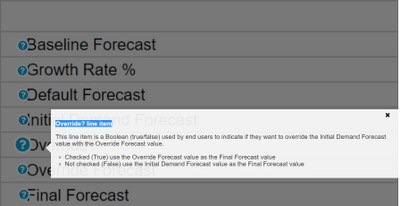# LVL 2 DEM03 Override? formulaHi Team,

Inserting formula for a boolean line item as:

IF Override? THEN Final Forecast = Override Forecast ELSE Final Forecast = Initial Demand ForecastTagged:

•Hi Kunal,

Override Line Item is a driver that indicates whether to take Initial Demand Forecast Value or Override Forecast Value and it is a manual input by end-user, not formula driven. Based on it the Final Forecast Value Line item will give the result

Write the formula in Final Forecast line item,

If Override is true, then take value from the Override Forecast Value line item else take it from the Initial Demand Forecast Value.

.

~Vignesh M

•Hi Vignesh,

I was looking for the solution for the same question too. My formula for DEM03 final forecast is IF Override? THEN Override Forecast ELSE Initial Demand Forecast

The data appears to be correct in the module, but when I copy this formula to the sprint 2 exam it is wrong. Would you please give some more advice?

I really appreciate it if you can help with this question.

•I am getting the same error. The formula is working in the module, but when i paste or type it in the text box, it shows INCORRECT answer.

Can anyone help!

•Hi @jingru and @ymandre ,

When building a formula you need to think which is the most possible occurrence of the condition: is it more likely to have overrides in the data or not? In this case overrides are not that frequent so you should switch your conditions starting with IF NOT Override? THEN .... ELSE ...

This is the best practice when building formulas so try it, it should work.

Elisabet

•Thank you Elisabet for explaining in a simple way. This is helpful!

•I had the same thing.  The module is calculating correctly so I can't figure out why it is marked wrong on the exam.

•So the formula I used was the IF NOT, the exam still states that it is wrong.  It is calculating in the model correctly though.